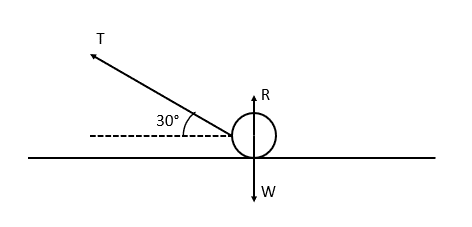# Newton's Laws

A LevelAQAEdexcelOCR

## Newton’s Laws

There are 3 Laws of Motion which are true for any system.

Make sure you are happy with the following topics before continuing.

A LevelAQAEdexcelOCR

## Newton’s First Law

A body will maintain a constant velocity (including rest), unless an external force is applied to that body.

A LevelAQAEdexcelOCR

## Newton’s Second Law

The resultant force on a body is equivalent to its mass, multiplied by its acceleration. Remember, the acceleration will be in the same direction as the resultant force. To be exact, we have the equation

$F = ma$

So, just as an example, in the Resolving Forces section, we’ve referenced the equation $W = mg$, relating weight to mass and the force of gravity. Weight is a force, and the value of $g$ is given as $9.8\text{ ms}^{-2}$, which is an acceleration.

A LevelAQAEdexcelOCR

## Newton’s Third Law

When two bodies collide, they exert forces upon each other which are equal in magnitude but opposite in direction.

You might have heard the phrase, “every action has an equal and opposite reaction”. So, let’s say you lean against a wall. Since you are exerting a force on the wall, the wall exerts an equal force back on you.

A LevelAQAEdexcelOCR

## Newton’s Laws in Vector Form

We can also apply $F = ma$ to a system with multiple components, by applying the formula to each component separately.

Example: say a $4\text{ kg}$ particle is travelling with an acceleration $\underline{a} = 2\textbf{i} + 3\textbf{j}$.

Then its force $F$ is given by $F = 4(2\textbf{i} + 3\textbf{j}) = 8\textbf{i} + 12\textbf{j}$.

A LevelAQAEdexcelOCR
A LevelAQAEdexcelOCR

## Example 1: Rising and Falling Objects

A bag of mass $6\text{ kg}$ is picked up with a force of $90\text{ N}$. Given that the only downward force is due to gravity, calculate its upward acceleration.

[2 marks]

First, we need to resolve our vertical forces. So, taking upwards to be the positive direction, we have

$90 - (6 \times 9.8) = 31.2\text{ N}$

Now, using Newton’s Second Law, $F = ma$, we have $31.2 = 6a$. By extension, $a = 5.2\text{ ms}^{-2}$.

A LevelAQAEdexcelOCR

## Example 2: Resolving in Perpendicular Directions

A particle is being pulled by a piece of string with a force of $17.32 \text{ N}$ at an angle of $30°$ to the horizontal. Given that there is no friction applied by the surface, and that the particle is accelerating horizontally at a rate of $3 \text{ ms}^{-2}$, what is the mass of the particle? What is the reaction force $R$?

[4 marks]Resolving horizontally: $T_H = 17.32\cos 30° = 15\text{ N}$

Since we have $F = ma$, we have $15 = 3m$, so the mass of the particle is $5\text{ kg}$.

Now, resolving vertically: $T_V + R = W$, we have $17.32\sin 30° + R = 5 \times 9.8$

Rearranging for $R$, we have $R = 49 - 17.32\sin 30° = 40.34\text{ N}$.

A LevelAQAEdexcelOCR

## Newton's Laws Example Questions

Using Newton’s Second Law, we have $F = 1.5 \times 9.8 = 14.7\text{ N}$.

Using Newton’s Third Law, we must have $R = W = 14.7\text{ N}$.

$F = ma$ gives $a = \dfrac{-0.1}{5} = -0.02\text{ ms}^{-2}$

From here,

$v = u + at$ gives $0 = 4.5 - 0.02t$

Then, after rearranging,

$t = \dfrac{4.5}{0.02} = 225\text{ s}$

$\underline{F} = m\underline{a}$

Then,

$\underline{F} = 0.5\begin{pmatrix}-9\\ -3\end{pmatrix} = \begin{pmatrix}-4.5\\ -1.5\end{pmatrix}$

A Level

A Level

A Level

## You May Also Like...### MME Learning Portal

Online exams, practice questions and revision videos for every GCSE level 9-1 topic! No fees, no trial period, just totally free access to the UK’s best GCSE maths revision platform.

£0.00

A Level

A Level

A Level

A Level